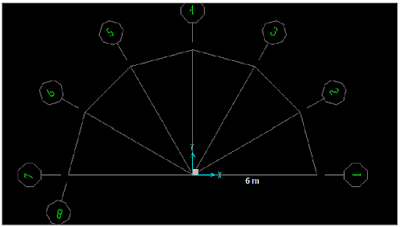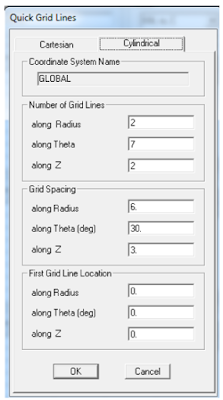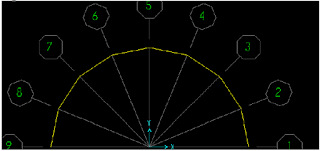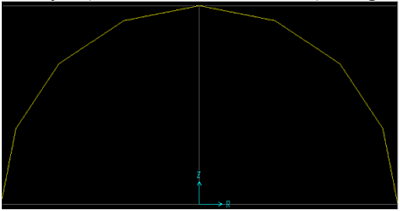### Introduction:

This is the last SAP2000 tutorial, at the end of this tutorial you'll be able to make a full structural analysis of any truss and areches and to display shear, moment and member forces diagrams as well.

### Solving 2D Trusses using blank template (Continued)

Example 1: draw and analyze the truss shown in figure 1 using SAP2000.
1- build the suitable grid system for the truss system (3 spacing in x- 1 spacing in z).
2- Draw the element of trusses using “moment releases” option as pinned instead of continuous. This will create moment releases at both ends (see figure 2).
3- Assign supports and loads and set self-weight multiplier to be zero to neglect self-weight.
4- Run analysis.
5-Display deformed shape and non-deformed shape at the same time. To do this see figure 3.
6- To display displacements at any point select it and then right click (see figure 4).
7- Change units to mm to get better displacement (see figure 5).

### Solving 2D Trusses using 2D truss template:

If user selects 2D truss template then awareness must be paid to end releases. Following steps are for choosing default vertical truss:

1- Choose 2D truss template as shown in figure 6.
2- End releases can be checked in two ways: A) - click on “set display options” then check releases as shown in figure 7.
Figure 8 indicates the members with moment releases. SAP suggestions can be modified manually by user.
B) Select any member than right click on it so you can display moment releases in the member selected as shown in figure 9.

### Trusses problems:

Problem #1:
Build this truss using SAP 2000 - see fig.10
Determine all the member forces
Identify the zero forces members
Problem #2:
Build this truss using SAP 2000 - see fig.11
Determine all the member forces
Identify the zero forces members
Problem #3:
Determine all the member forces
Identify the zero forces members
See fig.12
Problem #4:
Determine the force in members FG and DG of the truss shown in the figures below. State whether the members are in tension or compression.
Note: The reactions at the supports have been calculated.

### Creating arches using SAP2000:

Arches can easily be created using SAP if user learn how to build cylindrical systems. So learning how to build cylindrical systems is the first thing to be done.
Building a cylindrical grid is an easy process in SAP. To Define a cylindrical system follow these steps:

1- Open a new file; Choose Blank.
2- Right click ➥ Edit grid data ➥ high light “GLOBAL” ➥ Modify/show system ➥ Quick start.
3- To draw cylindrical grid choose cylindrical tab. Cylindrical systems are defined by radius (R) and Theta (ϴ) (see figure 14)

Quick start window to define cylindrical system[Fig.14]

Example 4:
Draw the grid line shown in figure 15.[Fig.15]

1- As mentioned before user needs to define R & ϴ, R is 6 m as shown in figure 10. User can determine ϴ simply by dividing 180 on 6 (6 is number of angles):

2- Input at quick start must be as shown in Fig. 15. Number of grid lines along radius can easily be determined using this relation: Number of grid lines along radius = No. of radiuses+1[Fig.16]

Exercise: Draw the cylindrical system shown in figure 17. Use R=6 m.[Fig.17]

Example: Build arch shown in figure 18 using SAP.[Fig.18]

1- SAP do not draw curves. Curves are represented as a small line segments connected to each others. This can be understood by looking at figure 19.[Fig.19]

2- The much lines you have the more accurate you are in the simulation of the arch.

3- This arch will be divided into 8 parts. You must understand that this number is not the only correct way to simulate the arch. You may use less or more lines. Now look at figure 20.[Fig.20]

4- Now user is going to create cylindrical system. Note that arch lies on xz plane while proposed grid will be drawn in xy plane.

5- Create a cylindrical system with the same input shown in figure 21.[Fig.21]

User must now have a cylindrical system exactly as the one shown in figure 22.[Fig.22]

6- Draw the arch in XY plane. User must have the same result as shown in figure 23.[Fig.23]

7- This step is about transferring the drawn arch from xy to xz. To do that, first select arch elements ➥ Edit ➥ replicate ➥ radial (see figure 24).[Fig.24]

After doing the previous step user will have same as shown in figure 25.[Fig.25]

8- Now change view to RZ (actually xz) to view the arch in 2D (see figure 26).[Fig.26]

9- Assign the supports to finish building the system (see figure 27).[Fig.27]